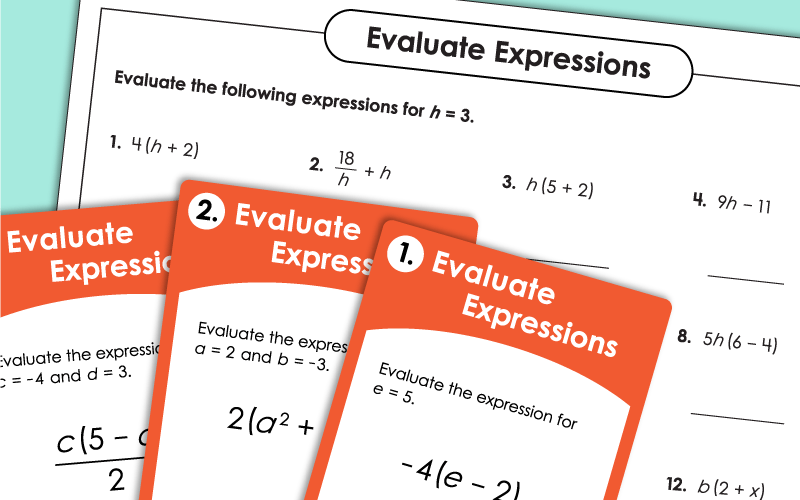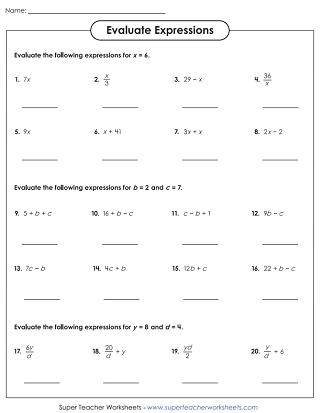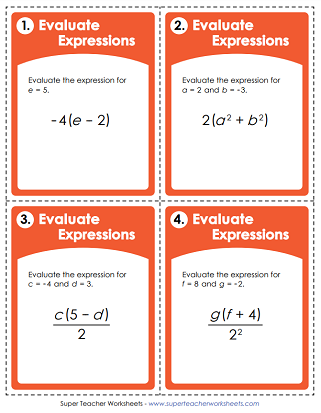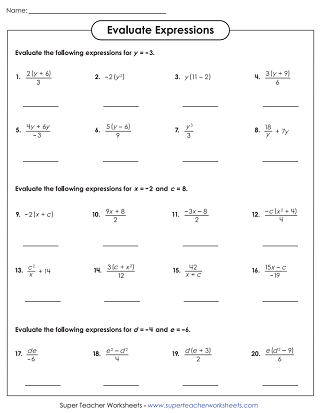# Evaluating Algebraic Expressions

On these printable worksheets, students will evaluate basic algebraic expressions with variables. These worksheets align with Common Core Standard 6.EE.2.## Basic Level:Positive Whole Numbers

Evaluate each algebraic expression. Values for the variables are given. This level does not include exponents, negative numbers, or parentheses.
Evaluate each expression; Substitute numbers for variables to solve. The basic level expressions do not include parentheses or exponents. On this worksheet, each expression has only one variable.
Students will evaluate expressions with one and two variables with values for the variables given.
Evaluate each expression and write the answers on the answer page. This file contains 30 task cards that can be used for classroom scavenger hunts, small group instruction, exit slips, and classroom games.

## Intermediate Level:Exponents & Parentheses

Evaluate each algebraic expression. Many problems in this level have parentheses and/or exponents.
Get more practice evaluating expressions with this worksheet. It includes fractions, parentheses, and exponents.
These intermediate-level expressions have exponents and parentheses. There are 30 task cards in the set. Perfect for document cameras, classroom scavenger hunts, peer tutoring, or small group instruction.

These advanced-level expressions include positive and negative numbers, as well as exponents and parentheses.
Practice evaluating more algebraic expressions. These include positive and negative numbers, exponents, and parentheses.
These advanced-level expressions have exponents, parentheses and negative numbers. 30 task cards in all.
More Pre-Algebra and Algebra Topics

Here you'll find out collection of algebra resources. We have activities for teaching equivalent expressions, inequalities, one and two-step equations, and more.

## Sample Worksheet ImagesMy Account
Site Information